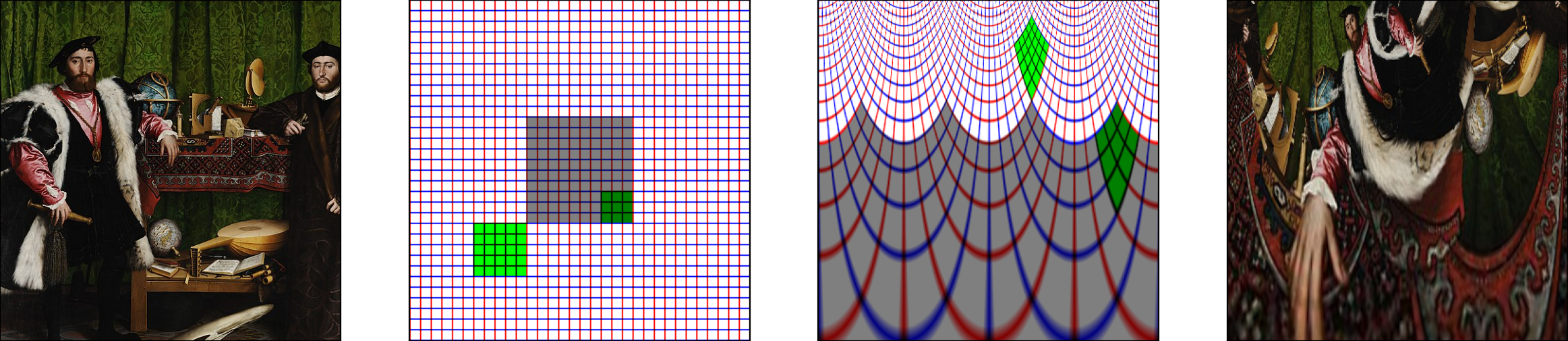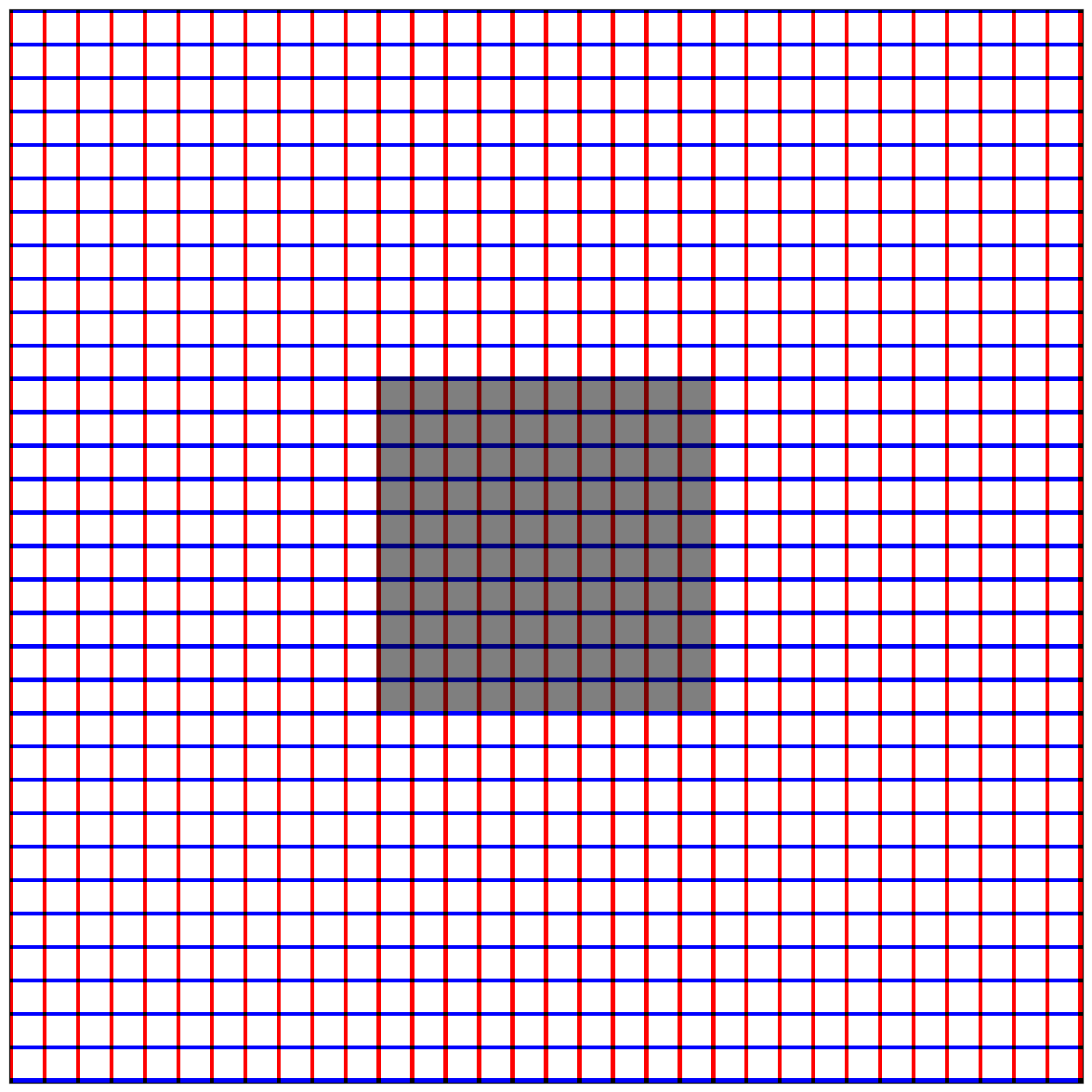# Implementing a retinotopic transform using `grid_sample` from pyTorch

## Implementing a retinotopic transform using `grid_sample` from `pyTorch`¶

The grid_sample transform is a powerful function which allows to transform any input image into a new topology. It is notably used in Spatial Transformer Networks for instance to learn CNN to be invariant to affine transforms. We used it recently in a publication What You See Is What You Transform: Foveated Spatial Transformers as a Bio-Inspired Attention Mechanism by Ghassan Dabane et al.

The use of `grid_sample` can b etedious and here, we show how to use it to create a log-polar transform of the image and create the following figure:A picture (extract from the painting "The Ambassadors" by Hans Holbein the Younger can be represented on a regular grid represented by vertical (red) and horizontal (blue) lines. Retinotopy transforms this grid, and in particular the area representing the fovea (shaded gray) is over-represented. Applied to the original image of the portrait, the image is strongly distorted and represents more finally the parts under the axis of sight (here the mouth).

Let's first initialize the notebook :

In :
```import os

import numpy as np
import torch
torch.set_printoptions(precision=3, linewidth=140, sci_mode=False)
import torch.nn.functional as F

import matplotlib.pyplot as plt
fig_width = 15

image_size = 257
```

### definition of the grid¶

Let's first define a first grid as a set of points defined in absolute coordinates between \$-1\$ and \$1\$, and define the corresponding meshgrid:

In :
```image_size_az, image_size_el = 360, 400
rs_ = torch.logspace(0, -4, image_size_az, base=2)
ts_ = torch.linspace(-torch.pi, torch.pi, image_size_el+1)[:-1]

grid_xs = torch.outer(rs_, -torch.cos(ts_))
grid_ys = torch.outer(rs_, torch.sin(ts_))
grid_xs.shape, grid_ys.shape
```
Out:
`(torch.Size([360, 400]), torch.Size([360, 400]))`

These are then formated in the right format to be used by the function:

In :
```center_x, center_y = 0., 0. # defines the fixation point's center in absolute coordinates
logPolar_grid = torch.stack((grid_xs-center_x, grid_ys-center_y), 2)
logPolar_grid = logPolar_grid.unsqueeze(0) # add batch dim
logPolar_grid.shape
```
Out:
`torch.Size([1, 360, 400, 2])`
In :
```logPolar_grid.min()
```
Out:
`tensor(-1.)`
In :
```# F.grid_sample?
```

### appication to a synthetic image¶

We define a synthetic image to illustrate the transform, it consists of white pixels, red verticals and blue horizontals, regularly spaced:

In :
```image_grid_size = 8
image_grid_tens = torch.ones((3, image_size, image_size)).float()
image_grid_tens[0:2, ::image_grid_size, :] = 0
image_grid_tens[1:3, :, ::image_grid_size] = 0
fovea_size = 5
image_grid_tens[:, (image_size//2-image_grid_size*fovea_size):(image_size//2+image_grid_size*fovea_size), (image_size//2-image_grid_size*fovea_size):(image_size//2+image_grid_size*fovea_size)] *= .5
image_grid_tens.shape, image_grid_tens.unsqueeze(0).shape
```
Out:
`(torch.Size([3, 257, 257]), torch.Size([1, 3, 257, 257]))`

to display it, we need to transform the torch format to a numpy / matplotlib compatible one, which can be first tested on a MWE (minimal working example) using `torch.movedim`:

In :
```torch.movedim(torch.randn(1, 2, 3), (0, 1, 2), (1, 2, 0)).shape
```
Out:
`torch.Size([3, 1, 2])`

this can be done on the image in a few lines:

In :
```image_grid = image_grid_tens.squeeze(0)
# swap from C, H, W (torch) to H, W, C (numpy)
image_grid = torch.movedim(image_grid, (1, 2, 0), (0, 1, 2))
image_grid = image_grid.numpy()
image_grid.shape
```
Out:
`(257, 257, 3)`

so that we can display the synthetic image:

In :
```fig, ax = plt.subplots(figsize=(fig_width, fig_width))
ax.imshow(image_grid)
ax.set_xticks([])
ax.set_yticks([])
fig.set_facecolor(color='white')
```Let's transform the image of the grid:

In :
```image_grid_ret_tens = F.grid_sample(image_grid_tens.unsqueeze(0).float(), logPolar_grid, align_corners=False, padding_mode='border')
image_grid_tens.shape, logPolar_grid.shape, image_grid_ret_tens.shape
```
Out:
```(torch.Size([3, 257, 257]),
torch.Size([1, 360, 400, 2]),
torch.Size([1, 3, 360, 400]))```

and transform it back to numpy:

In :
```image_grid_ret_tens = image_grid_ret_tens.squeeze(0)
# swap from C, H, W (torch) to H, W, C (numpy)
image_grid_ret_tens = torch.movedim(image_grid_ret_tens, (1, 2, 0), (0, 1, 2))
image_grid_ret = image_grid_ret_tens.numpy()
image_grid_ret /= image_grid_ret.max()
image_grid_ret.shape
```
Out:
`(360, 400, 3)`

to then display the retinotopic transform of the grid image:

In :
```fig, ax = plt.subplots(figsize=(fig_width, fig_width))
ax.imshow(image_grid_ret)
ax.set_xticks([])
ax.set_yticks([])
fig.set_facecolor(color='white')
```